$$\newcommand{\id}{\mathrm{id}}$$ $$\newcommand{\Span}{\mathrm{span}}$$ $$\newcommand{\kernel}{\mathrm{null}\,}$$ $$\newcommand{\range}{\mathrm{range}\,}$$ $$\newcommand{\RealPart}{\mathrm{Re}}$$ $$\newcommand{\ImaginaryPart}{\mathrm{Im}}$$ $$\newcommand{\Argument}{\mathrm{Arg}}$$ $$\newcommand{\norm}{\| #1 \|}$$ $$\newcommand{\inner}{\langle #1, #2 \rangle}$$ $$\newcommand{\Span}{\mathrm{span}}$$

# 2.1: Dots and Boxes

•• Contributed by Michelle Manes
• Professor (Mathematics) at University of Hawaii
$$\newcommand{\vecs}{\overset { \rightharpoonup} {\mathbf{#1}} }$$ $$\newcommand{\vecd}{\overset{-\!-\!\rightharpoonup}{\vphantom{a}\smash {#1}}}$$$$\newcommand{\id}{\mathrm{id}}$$ $$\newcommand{\Span}{\mathrm{span}}$$ $$\newcommand{\kernel}{\mathrm{null}\,}$$ $$\newcommand{\range}{\mathrm{range}\,}$$ $$\newcommand{\RealPart}{\mathrm{Re}}$$ $$\newcommand{\ImaginaryPart}{\mathrm{Im}}$$ $$\newcommand{\Argument}{\mathrm{Arg}}$$ $$\newcommand{\norm}{\| #1 \|}$$ $$\newcommand{\inner}{\langle #1, #2 \rangle}$$ $$\newcommand{\Span}{\mathrm{span}}$$ $$\newcommand{\id}{\mathrm{id}}$$ $$\newcommand{\Span}{\mathrm{span}}$$ $$\newcommand{\kernel}{\mathrm{null}\,}$$ $$\newcommand{\range}{\mathrm{range}\,}$$ $$\newcommand{\RealPart}{\mathrm{Re}}$$ $$\newcommand{\ImaginaryPart}{\mathrm{Im}}$$ $$\newcommand{\Argument}{\mathrm{Arg}}$$ $$\newcommand{\norm}{\| #1 \|}$$ $$\newcommand{\inner}{\langle #1, #2 \rangle}$$ $$\newcommand{\Span}{\mathrm{span}}$$

Here are some dots; in fact there are nine of them: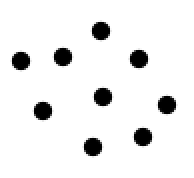We’re going to a play an “exploding dots” game. Here’s the only rule for the game:

The 1←2 Rule

Whenever there are two dots in single box, they “explode,” disappear, and become one dot in the box to the left.

Example $$\PageIndex{1}$$: Nine dots 1←2 in the system

We start by placing nine dots in the rightmost box.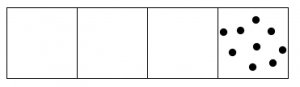Two dots in that box explode and become one dot in the box to the left.Once again, two dots in that box explode and become one dot in the box to the left.We do it again!Hey, now we have more than two dots in the second box, so those can explode and move!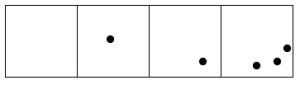And the rightmost box still has more than two dots.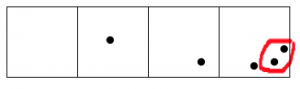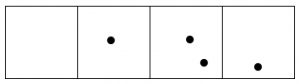Keep going, until no box has two dots.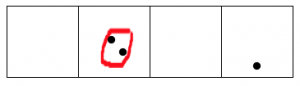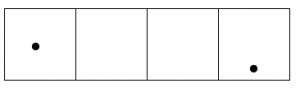After all this, reading from left to right we are left with one dot, followed by zero dots, zero dots, and one final dot.

The 1←2 code for nine dots is: 1001.

On Your Own. Here’s a diagram showing what happens for seven dots in a 1←2 box. Trace through the diagram, and circle the pairs of dots that “exploded” at each step.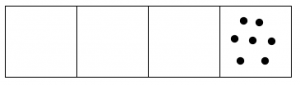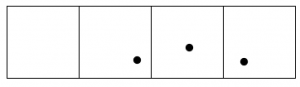The 1←2 code for seven dots is: 111.

Problem 1

Note: In solving this problem, you don’t need to draw on paper; that can get tedious! Maybe you could use buttons or pennies for dots and do this by hand.

1. Draw 10 dots in the right-most box and perform the explosions. What is the 1←2 code for ten dots?
2. Find the 1←2 code for eighteen dots.
3. What number of dots has 1←2 code 101?

Think / Pair / Share

After you worked on the problem, compare your answer with a partner. Did you both get the same code? Did you have the same process?